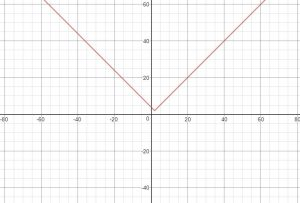# GATE | GATE CS Mock 2018 | Set 2 | Question 10

Choose the correct expression for f(x) given in the graph.(A) f(x) = 2 – |x + 2|
(B) f(x) = 2 + |x − 2|
(C) f(x) = 2 – |x − 2|
(D) f(x) = 2 + |x + 2|

Explanation: Verify using some random values.
Option (B) f(x) = 2 + |x − 2| is correct option.

Quiz of this Question

My Personal Notes arrow_drop_up
Article Tags :

Be the First to upvote.

Please write to us at contribute@geeksforgeeks.org to report any issue with the above content.